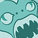# QlikView App Dev

Discussion Board for collaboration related to QlikView App Development.

Announcements
An issue has been identified on Qlik Cloud hub, please visit our Status Update Page for details: GET THE LATEST
cancel
Showing results for
Did you mean:Contributor III

## Current and Previous Quarter calculations

Hi all,

I am creating a Quarter field with the following script:

'Q' & ceil(right([Acc_Calendar.Acc Period],2)/3) as Quarter

How do i then create a vCurrentQuarter and vPreviousQuarter ?

7 RepliesMaster

Hi Kieron,

Consider a separate numeric QuarterID field such as

ceil(right([Acc_Calendar.Acc Period],2)/3)  as QuarterID

or you can try defining your quarter as a Dual:

Dual('Q' & ceil(right([Acc_Calendar.Acc Period],2)/3) , ceil(right([Acc_Calendar.Acc Period],2)/3) ) as Quarter

Cheers

AndrewContributor III
Author

Thanks Andrew,

That gives me a numeric value for each quarter, what is the logic then to get current quarter (or previous quarter). To get Current week i have used: let vCurrent_week = num(week(today()), '00');

To get current year week i have used: let vCurrent_yearweek = weekyear(today()) & num(week(today()), '00');

But i'm confused about current quarter.

Thanks,

KieronMaster

Hi Keiron,

In the UI \$(=max(QuarterID)) will give the current QuarterID according to the selections and \$(=-1+max(QuarterID)) will give the previous.

Cheers

AndrewCreator II

You can try below one

vCurrent_Quarter=   Year={"\$(=GetFieldSelections(Quarter))"}

vPrevious_Quarter = Year={"\$(=GetFieldSelections(Quarter)-1)"}

sum({<\$(=vCurrent_Quarterr)>}Sales)

sum({<\$(=vvPrevious_Quarter)>}Sales)Creator II

You can try below one

vCurrent_Quarter=   Quarter={"\$(=GetFieldSelections(Quarter))"}

vPrevious_Quarter = Quarter={"\$(=GetFieldSelections(Quarter)-1)"}

sum({<\$(=vCurrent_Quarterr)>}Sales)

sum({<\$(=vvPrevious_Quarter)>}Sales)Contributor III
Author

Hi devendarlakkyreddy‌ where should i try create those? in the script editor or in my calculation ?

Thanks,

KieronCreator II

by using Quarter field in the front end create a variable , then use calculation in the chartCommunity Browser